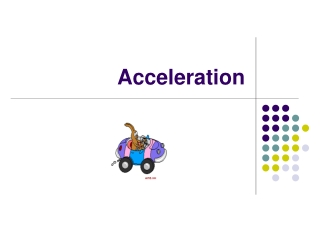DownloadDownload PresentationAcceleration

# Acceleration

Télécharger la présentation## Acceleration

- - - - - - - - - - - - - - - - - - - - - - - - - - - E N D - - - - - - - - - - - - - - - - - - - - - - - - - - -
##### Presentation Transcript

1. Acceleration

2. The pitcher throws. The ball speeds toward the batter. Off the bat it goes. It’s going, going, gone! A home run! • Before landing, the ball went through several changes in motion. It sped up in the pitcher’s hand, and lost speed as it traveled toward the batter. The ball stopped when it hit the bat, changed direction, sped up again, and eventually slowed down. Most examples of motion involve similar changes. In fact, rarely does any object’s motion stay the same for very long.

3. What Is Acceleration? • Suppose you are a passenger in a car stopped at a red light. When the light changes to green, the driver steps on the accelerator. As a result, the car speeds up, or accelerates. In everyday language, acceleration means “the process of speeding up.” • Acceleration has a more precise definition in science. Scientists define acceleration as the rate at which velocity changes. Recall that velocity describes both the speed and direction of an object. A change in velocity can involve a change in either speed or direction—or both. In science, acceleration refers to increasing speed, decreasing speed, or changing direction.

4. Increasing Speed • Whenever an object’s speed increases, the object accelerates. A softball accelerates when the pitcher throws it, and again when a bat hits it. A car that begins to move from a stopped position or speeds up to pass another car is accelerating. People can accelerate too. For example, you accelerate when you coast down a hill on your bike.

5. Decreasing Speed • Just as objects can speed up, they can also slow down. This change in speed is sometimes called deceleration, or negative acceleration. For example, a softball decelerates when it lands in a fielder’s mitt. A car decelerates when it stops at a red light. A water skier decelerates when the boat stops pulling.

6. Changing Direction • Even an object that is traveling at a constant speed can be accelerating. Recall that acceleration can be a change in direction as well as a change in speed. Therefore, a car accelerates as it follows a gentle curve in the road or changes lanes. Runners accelerate as they round the curve in a track. A softball accelerates when it changes direction as it is hit.

7. Many objects continuously change direction without changing speed. The simplest example of this type of motion is circular motion, or motion along a circular path. For example, the seats on a Ferris wheel accelerate because they move in a circle.

8. Calculating Acceleration • Acceleration describes the rate at which velocity changes. If an object is not changing direction, you can describe its acceleration as the rate at which its speed changes. To determine the acceleration of an object, you must calculate the change in speed during each unit of time. This is summarized by the following formula. • Acceleration = (Final speed – Initial speed) Time

9. If speed is measured in meters/second and time is measured in seconds, the SI unit of acceleration is meters per second per second, or m/s2. Suppose speed is measured in kilometers per hour and time is measured in hours. Then the unit of acceleration is kilometers per hour per hour, or km/h2.

10. To understand acceleration, imagine a small airplane moving down a runway. Figure 10 shows the airplane’s motion after each of the first five seconds of its acceleration. To calculate the average acceleration of the airplane, you must first subtract the initial speed of 0 m/s from the final speed of 40 m/s. Then divide the change in speed by the time, 5 seconds.

11. Figure 10

12. The airplane accelerates at a rate of 8 m/s2. This means that the airplane’s speed increases by 8 m/s every second. Notice in Figure 10 that, after each second of travel, the airplane’s speed is 8 m/s greater than it was the previous second.

13. Practice Problems • Calculating Acceleration A falling raindrop accelerates from 10 m/s to 30 m/s in 2 seconds. What is the raindrop’s average acceleration? • (2) Calculating Acceleration A certain car can accelerate from rest to 27 m/s in 9 seconds. Find the car’s average acceleration.